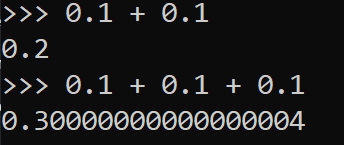# Binary Coded Decimal

## What is Binary Coded Decimal

Binary Coded Decimal is a form of binary representation where each denary digit is converted individually into binary, rather than the whole number being converted as a large number.

### Example 1 – the number 42

Normally this number would be converted directed to binary as 00101010  as 8 bit binary.

 128 64 32 16 8 4 2 1 0 0 1 0 1 0 1 0

However in binary coded decimal we would convert the number 4 to binary and the number 2 to binary, then simply concatenate(combine) the 2 numbers together

Binary 4

 8 4 2 1 0 1 0 0

Binary 2

 8 4 2 1 0 0 1 0

Therefore 42 in BCD binary would be 01000010

## Why use BCD?

### Why use Binary Coded Decimal?

Binary coded decimal has 3 main advantages

• It is easier for a human to read large binary numbers than if the number is in pure binary
• It is simpler to create hardware than only counts in decimal and uses basic calculation (e.g. digital clocks, simple handheld calculators)
• Binary coded decimal removes the problem of floating point rounding errors, which makes is very useful for handling financial transactions.

## BCD Clock Demo

### Binary Coded Decimal Clock Demonstration Project

The Scratch project for this clock can be found here

## Financial systems

### Financial Systems

In binary systems decimals numbers are stored using a floating point representation. When representing fractions of a whole some numbers cannot be stored accurately using binary, due to the fractions not fitting perfectly within a binary base 2 system. This results in a floating point rounding error. In financial systems rounding errors are unacceptable and must be dealt within. For this reason binary coded decimal is used instead.

Floating point rounding exampleHere is a great example of a floating point binary rounding error. This kind of error is not acceptable in financial transactions.